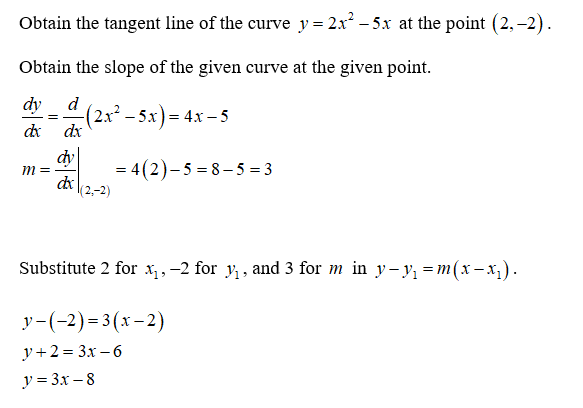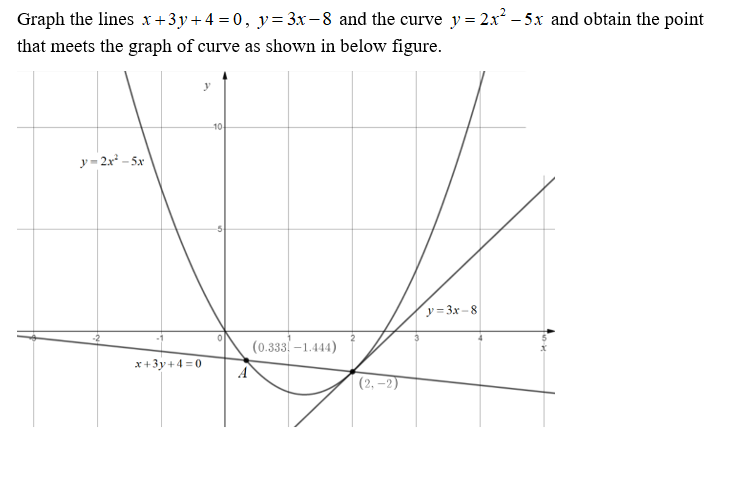# The equation x+3y+4=0 is the normal line to the curve y=2x2-5x at (2, -2). Given that the line meets the graph again at A, give the coordinates of A.

Question
1 views

The equation x+3y+4=0 is the normal line to the curve y=2x2-5x at (2, -2). Given that the line meets the graph again at A, give the coordinates of A.

check_circle

Step 1Step 2...

### Want to see the full answer?

See Solution

#### Want to see this answer and more?

Solutions are written by subject experts who are available 24/7. Questions are typically answered within 1 hour.*

See Solution
*Response times may vary by subject and question.
Tagged in